Oil Reserves Uncertainties

Introduction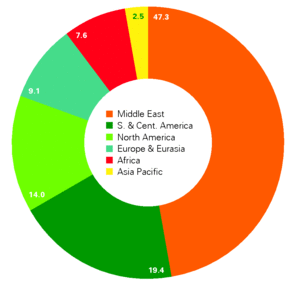Fig. 1: World's 2015 Proved Oil Reserves, as reported by British Petroleum, broken down by geographical region.  The units are percent. The total 2015 world reserve reported by BP was 1.70 × 1012 bbls (2.38 × 1011 tonnes).  (Courtesy of British Petroleum.)

Estimating oil reserves is one of the most important and most influential tasks petroleum engineers perform. Reserves are defined as the petroleum quantities that are commercially recoverable by application of development projects under defined conditions. Resources are different than reserves. Resources are defined as all petroleum quantities naturally occurring in a reservoir, discovered and undiscovered, both recoverable and unrecoverable.  (See Fig. 1.) Estimating the amount of reserves in a field is not a trivial task and is important for companies because it allows them to keep track of how much oil/gas is still left in their prospects and to update their investors about their predictions of future gains/losses. The task is also very influential because increasing/decreasing that number by large companies/countries can have deep consequences on the world's markets. Estimating reserves always encompasses some degree of uncertainty.  This uncertainty stems mainly from the lack of geological data (i.e. porosity and permeability). The uncertainty level fades away as the field is further developed and more data/feedback is collected from the field but never goes away. Depending on the available data, different methods can be applied to estimate reserves. For a new field, the volumetric method provides the most straightforward analysis by using data from nearby fields. As the field gets older and more production data is available, decline curve analysis provides a more robust option. Lastly, as we collect more geological data and production data, reservoir simulation models are the most suitable option to perform these reserves estimation studies. In this report, I will introduce each of these methods and then I will perform a simple Monte Carlo simulation using the volumetric method to show the level of uncertainty in reserves estimation.

Methods

1. Volumetric Method

Early in the life of a field, when geological data are available only from exploration wells and/or from analogous fields nearby, one would choose the volumetric method to estimate reserves. The method uses this simple volumetric relation:

Reserves = A h φ (1 - Swi)/Bo × RF

where A is the reservoir's area in (m2), h is the thickness in (m), φ is the porosity, Swi is initial/irreducible water saturation, Bo is the oil formation volume factor in (m3/m3), and RF is the recovery factor. This method will be used in later in another section to perform Monte Carlo simulation and study the uncertainty in oil reserves. Then, we will discuss the uncertainty in each parameter.

2. Decline Curve Analysis

As the reservoir begins to mature and more production data becomes available, decline curve analysis becomes a better method to estimate reserves that a lesser degree of uncertainty. Decline curve analysis uses empirical equations that models how the flow rate changes with time assuming a certain decline rate.  A major assumption here is that the most dominant past behavior will govern the future behavior of the well's/field's performance. Obviously, this is not necessarily true but works in many cases. It could also yield reasonable results when more wells are lumped together.  We note that the decline curve analysis ignores any geological information from the field and, therefore, could give very unreasonable results in some cases (e.g. in unconventional wells or fractured reservoirs). The two main models as pointed out by Rustem are: 

 Exponential model: q(t) = qi exp(-Dit) Hyperbolic model: q(t) = qi (1 + bDit)-1/b

where qi is the initial flow rate in (stm3/day), Di is the initial decline rate, and b is the degree of curvature of the line in the hyperbolic model.

3. Numerical Reservoir Simulation

Another method that is capable of estimating reserves to a lesser degree of uncertainty than the above two methods is numerical reservoir simulation. A reservoir simulator numerically solves mass and energy conservation equations.  The equations are discretized on a 3D grid. Each cell in the grid has different porosity and permeability values and the flow between blocks are computed numerically. The fluid flow is assumed to be governed by Darcy's law. The reservoir simulation model usually uses stochastically estimated values for the geological parameters such as porosity and permeability using geostatistical methods. The common practice does not eliminate uncertainty all at once because there is still large uncertainty in the geological data of the reservoir. In essence, two geological realizations of the reservoir can produce the same or similar results. The current practice of populating these goestatistical parameters does not take this into account. reservoir simulator.

Monte Carlo Simulation on the Volumetric Method

In this section, we use the Monte Carlo technique to obtain the uncertainty of the reserves estimate using the volumetric method. In the Monte Carlo technique, each input variable is given a probability distribution, then samples are randomly drawn from these pre-defined distributions and combined to obtain a possible output or realization.  The first step is, then, to define distributions for each of the input variables in the volumetric equation. Table 1 lists the input parameters for the Monte Carlo simulation performed here. Fig. 2 shows the probability density functions used for the input variables.

Property Symbol Distribution Mean Standard deviation Lower limit Upper limit Peak
Area (m2) A Truncated normal 663684 161874 121406 841746 --
Thickness (m) h Truncated normal 6 3 3 9 --
Porosity (%) φ Truncated normal 10 5 2 30 --
Water Saturation (%) Swi Triangular -- -- 20 35 30
Volume Factor (m3/m3) Bo Uniform -- -- 1.2 1.4 --
Recovery Factor (%) RF Truncated normal 30 7.5 10 50 --
Table 1: Monte Carlo input parameters. The symbols are defined as per the volumetric model. The probability distributions are shown explicitly in Fig. 2.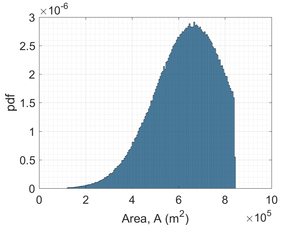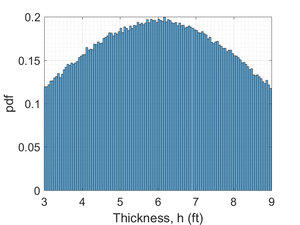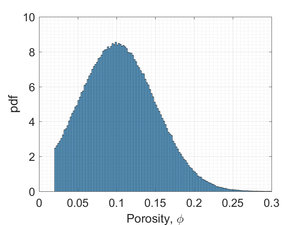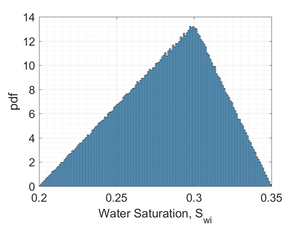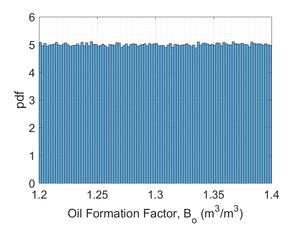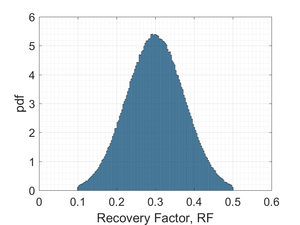Fig. 2: Probability distributions characterized by the parameters in Table 1. (Source: M. Almajid)

The input parameters ranges above were chosen to mimic the characteristics of fields in a district in Magyarstan as shown by Harbaugh et al.  The parameters were adjusted a bit to be more realistic. It is assumed that the area, thickness, porosity, and recovery factor are normally distributed. The distributions of these variables were truncated to ensure physical limits are met. For example, the porosity is not allowed to have negative values. The initial water saturation is assumed to have a triangular distribution, which is a valid assumption as the initial water in the reservoir does not vary significantly from one place to another. Lastly, the oil formation volume factor is assumed to be uniformly distributed because this information is usually know with good certainty from lab measurements.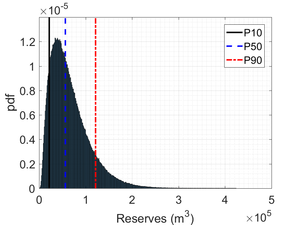Fig. 3: Reserves probability density function output. (Source: M. Almajid)

The Monte Carlo simulation was implemented in Matlab and the number of samples was chosen to be 1,000,000. The result is a probability density function of the reserves as shown in Fig. 3. On the same figure, we note the p10, p50, and p90 values. These are all possible outcomes. Usually, the reported values are the p50 values but it's not guaranteed that each company will use this value as their official number of the reserves. We note that the difference between the p90 and p10 values is about 82%. This is a huge number and kind of puts in perspective the uncertainty in estimating the oil reserves in practice.

Conclusion

In this short report, we defined what reserves are and differentiated them from resources. We also discussed why estimating reserves is very important for a company/country. Later, we briefly reviewed the various methods used to estimated reserves and when each one will be suitable. Lastly, a simple Monte Carlo simulation was performed on the volumetric method to determine the amount of uncertainty in oil reserves estimation. It was found that the difference between the p90 and p10 values is the order of 80%. This is a significant amount that highlights the amount of uncertainty faced in the oil industry when estimating oil reserves.

© Muhammad Almajid. The author grants permission to copy, distribute and display this work in unaltered form, with attribution to the author, for noncommercial purposes only. All other rights, including commercial rights, are reserved to the author.

References

 "Petroleum Resources Management System," Society of Petroleum Engineers, January (2008).

 R. Zaydullin, "Oil Recovery Prediction," Physics 240, Stanford University, Fall 2013.

 J. W. Harbaugh, J. C. Davis, and J. Wendebourg, Computing Risk for Oil Prospects: Principles and Programs (Pergamon, 1995).

 "BP Statistical Review of World Energy 2016," British Petroleum, June 2016.